# How to write a dot product(a • b) in LaTeX?

Mathematically, you see a lot of multiplications where dot symbol are used instead of cross symbol.

Some of these multiplications are known as vector dot product. Vector products are always represented by dot symbol between two or more vectors.

So, to represent this dot product with the help of latex, you need to take the help of \cdot command. And this \cdot command will always return the dot symbol.

\documentclass{article}
\begin{document}
$$\vec{p} \cdot \vec{q}$$
\end{document}

Output :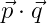And notice the output above. Here the \vec{} command is used for the vector arrow sign.

You can denote a vector by converting a variable or text to bold font style without using arrows.

\documentclass{article}
\begin{document}
$$\textbf{p} \cdot \textbf{q}$$
\end{document}


Output :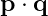So, to make a particular text bold, you need to call the \textbf{} command.

## Dot product in the form of Cosθ in LaTeX

The result of the dot product is expressed in the form of cos-theta which is a scalar.

You need to use both cos and theta commands simultaneously for this cos theta symbol. And you don’t have to pass any argument between the two commands.

\documentclass{article}
\begin{document}
$$\vec{p} \cdot \vec{q}=\left | \vec{p} \right |\left | \vec{q} \right |\cos\theta$$
\end{document}

Output :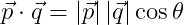However, for the absolute value of the vector, the vector has to be passed through the \left| and \right| commands.

## Result of dot product in the form of position vector

If you know the position of two vectors, you can easily define a dot product between them.

And in this case you need to know how to define position vectors with the help of latex.

\documentclass{article}
\begin{document}
$$\vec{p}=(x_{1}\hat{i}+y_{1}\hat{i}+z_{1}\hat{i})$$
$$\vec{q}=(x_{2}\hat{i}+y_{2}\hat{i}+z_{2}\hat{i})$$
$$\vec{p}\cdot\vec{q}=x_{1}x_{2}+y_{1}y_{2}+z_{1}z_{2}$$
\end{document}

Output :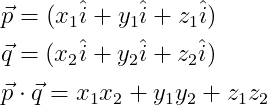So, look at that program above. Here a unit vector is denoted by a cap on a single letter.

And for this you have to use the \hat command and pass the letter (i,j,k) on which you want to see the hat(Â) symbol as an argument.

## Result of dot product in the form of Matrix Product

You will notice many science books or research papers where dot products are written as the product of column and row matrix.

So, if we take two vectors, one has to be written in the form of row matrix and the other in the form of column matrix.

So if you multiply the matrix between them, the result of the dot product will return.

\documentclass{article}
\usepackage{amsmath}
\begin{document}
$$\vec{p}\cdot\vec{q}= \begin{pmatrix} x_{1} & y_{1} & z_{1} \end{pmatrix} \begin{pmatrix} x_{2}\\ y_{2}\\ z_{2} \end{pmatrix}$$
\end{document}


Output :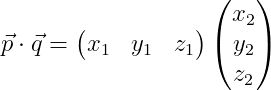## Vector triple Product in latex

A triple product is a combination of a dot product and a cross product.

\documentclass{article}
\begin{document}
$\vec{a}\cdot(\vec{b}\times\vec{c}\,)$
\end{document}

Output :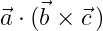You can use the \times command for cross marks. And the s command will create a light space between the p-vector and the close bracket.#### Md Jidan Mondal

LaTeX expert with over 10 years of experience in document preparation and typesetting. Specializes in creating professional documents, reports, and presentations using LaTeX.